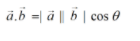Vectors homework Help at TutorEye

Vectors:

Vector is the representation of an object that comprises both magnitude and direction. It is a geometrical representation, which constitutes length of ray, that represents magnitude and arrow in the direction. It finds application in representing force or motion of objects along with the direction in classical mechanics for solving the static and kinematic problems.

Vectors Sample Questions:

Question 1: The vectors which meet at one point, but their line of action do not lie in the plane are called

a) Coplanar, non-concurrent vectors
b) Non-coplanar, concurrent vectors
c) Non-coplanar, non-concurrent vectors
d) Intersecting vectors

Explanation: Given,
Two or more force vectors that act at the same point

Question 2: The resultant of two force vectors P and Q inclined at angle θ will be inclined at the following angle with respect to P?

a)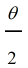(b)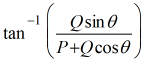(c)(d)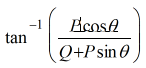Answer:  (b)Explanation: In triangle ORS
Given, parallelogram law of vectors,

Question 3: The angle between two force vectors when the resultant is maximum and minimum respectively are

(a)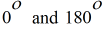(b)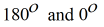(c)(d)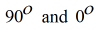Answer: (a)Explanation: Given,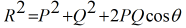Question 4:  The resultant of two force vectors, One of which is double the other is 260N. If the direction of the larger force vector is reversed and the other remains unaltered, the resultant reduces to 180N. The magnitude of the force vector and the angle between the force vectors are:

a) 150, 300, 70
b) 100, 200, 63.89
c) 50, 100, 40
d) 70, 140, 50

Explanation: Given,

Let, smaller force vector = P

Question 5:  lf the maximum and minimum resultant forces of two forces acting on a particle are 40 kN and 10 kN respectively, then the two forces in question would be?

a) 25 KN, 15 KN
b) 20 KN, 20 KN
c) 20 KN, 10 KN
d) 20 KN, 50 KN

Answer: a) 25 KN, 15 KN

Explanation: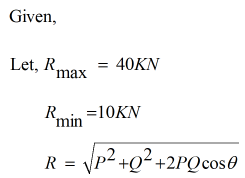Question 6: A triangle is formed by the following vectors. Find the va;ue of lambda.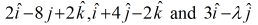a) -2               b) -12

c) 12               d) 2

Explanation:  According to the addition law, In triangle

Question 7: If a + b and a.b = 0 forms an angle 30°, Then

(a)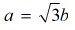(b)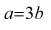(c)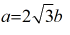(d)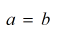Answer: (a)Explanation: Given,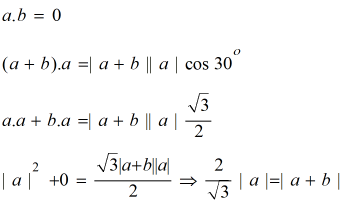Question 8: If |a| = |b| = 1 and |a+b| = 1, then the value of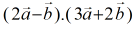a) 5/2        b) 3/2

c) 7/2        d) 9/2

Explanation:  Given,

|a+b| = 1

Question 9: Given vectors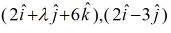. For what value of lambda, it is a right angle triangle.

a) 1/2         b) 3/2

c) 4/3         d) 1/4

Explanation:  For right angled, dot product to be zero.

Question 10: The angle between vectors A and B  is 60° and magnitudes are 2 and 3 respectively. Find A.B

a)  2        b) 3

c)  4        d) 1